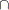INTER Aggregation Expression

INTER { index-set } set-expression

The INTER aggregation operator evaluates the set-expression for each member of the index set index-set. The result is the set that contains the intersection of the set of values that were returned by the set-expression for each member of the index set. An empty index set causes an expression evaluation error.

The following statements use the INTER aggregation operator to compute the value of {1,2,3,4}{2,3,4,5}{3,4,5,6}:

```proc optmodel;
put (inter{i in 1..3} i..i+3); /* outputs {3,4} */
```